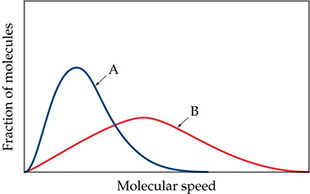# Problem: Consider the following drawing.If curves A and B refer to two different gases, He and O2 at the same temperature, which curve corresponds to He?

🤓 Based on our data, we think this question is relevant for Professor Lapeyrouse's class at UCF.

###### FREE Expert Solution

We are asked which curve corresponds to He.

Molar mass He = 4.003 g/mol

Molar mass O2 = 32 g/mol###### Problem Details

Consider the following drawing.If curves A and B refer to two different gases, He and O2 at the same temperature, which curve corresponds to He?